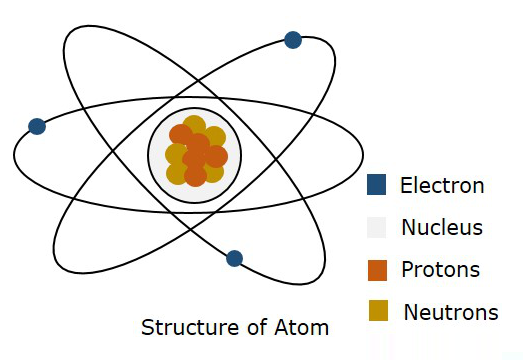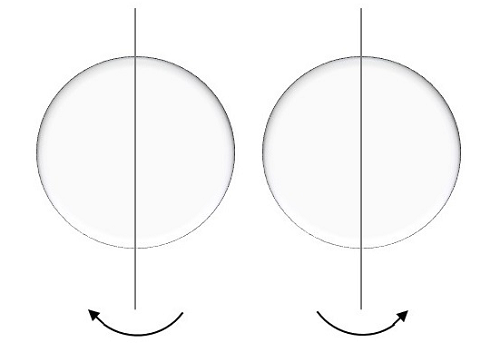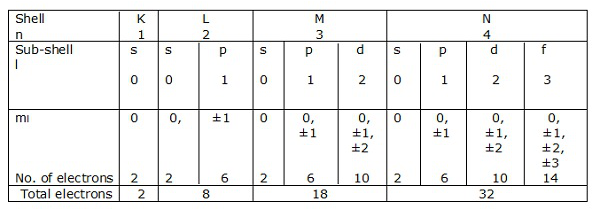# Basic Electronics – Materials

Matter is made up of molecules which consists of atoms. According to Bohrs theory, âthe atom consists of positively charged nucleus and a number of negatively charged electrons which revolve round the nucleus in various orbitsâ. When an electron is raised from a lower state to a higher state, it is said to be excited. While exciting, if the electron is completely removed from the nucleus, the atom is said to be ionized. So, the process of raising the atom from normal state to this ionized state is called as ionization.

The following figure shows the structure of an atom.According to Bohrs model, an electron is said to be moved in a particular Orbit, whereas according to quantum mechanics, an electron is said to be somewhere in free space of the atom, called as Orbital. This theory of quantum mechanics was proven to be right. Hence, a three dimensional boundary where an electron is probable to found is called as Atomic Orbital.

## Quantum Numbers

Each orbital, where an electron moves, differs in its energy and shape. The energy levels of orbitals can be represented using discrete set of integrals and half-integrals known as quantum numbers. There are four quantum numbers used to define a wave function.

### Principal Quantum number

The first quantum number that describes an electron is the Principal quantum number. Its symbol is n. It specifies the size or order (energy level) of the number. As the value of n increases, the average distance from electron to nucleus also increases, as well, the energy of the electron also increases. The main energy level can be understood as a shell.

### Angular Momentum Quantum number

This quantum number has l as its symbol. This l indicates the shape of the orbital. It ranges from 0 to n-1.

l = 0, 1, 2 â¦n-1

For the first shell, n = 1.

i.e., for n-1, l = 0 is the only possible value of l as n = 1.

So, when l = 0, it is called as S orbital. The shape of S is spherical. The following figure represents the shape of S.If n = 2, then l = 0, 1 as these are the two possible values for n = 2.

We know that it is S orbital for l = 0, but if l = 1, it is P orbital.

The P orbital where the electrons are more likely to find is in dumbbell shape. It is shown in the following figure.### Magnetic Quantum number

This quantum number is denoted by ml which represents the orientation of an orbital around the nucleus. The values of ml depend on l.

$$m_{l}= \int (-l\:\:to\:+l)$$

For l = 0, ml = 0 this represents S orbital.

For l = 1, ml = -1, 0, +1 these are the three possible values and this represents P orbital.

Hence we have three P orbitals as shown in the following figure.### Spin Quantum number

This is represented by ms and the electron here, spins on the axis. The movement of the spinning of electron could be either clockwise or anti-clockwise as shown here under.The possible values for this spin quantum number will be like,

$$m_{s}= +\frac{1}{2}\:\:up$$

For a movement called spin up, the result is positive half.

$$m_{s}= -\frac{1}{2}\:\:down$$

For a movement called spin down, the result is negative half.

These are the four quantum numbers.

## Pauli Exclusion Principle

According to Pauli Exclusion Principle, no two electrons in an atom can have the same set of four identical quantum numbers. It means, if any two electrons have same values of n, s, ml (as we just discussed above) then the l value would definitely be different in them. Hence, no two electrons will have same energy.

### Electronic shells

If n = 1 is a shell, then l = 0 is a sub-shell.

Likewise, n = 2 is a shell, and l = 0, 1 is a sub-shell.

Shells of electrons corresponding to n = 1, 2, 3â¦.. are represented by K, L, M, N respectively. The sub-shells or the orbitals corresponding to l = 0, 1, 2, 3 etc. are denoted by s, p, d, f etc. respectively.Let us have a look at the electronic configurations of carbon, silicon and germanium (Group IV â A).It is observed that the outermost p sub-shell in each case contains only two electrons. But the possible number of electrons is six. Hence, there are four valence electrons in each outer most shell. So, each electron in an atom has specific energy. The atomic arrangement inside the molecules in any type of substance is almost like this. But the spacing between the atoms differ from material to material.